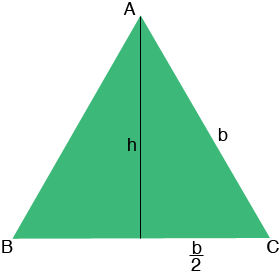SEARCH HOMEMath Central Quandaries & QueriesQuestion from michael, a parent: my daughter has been given a task and I'm stuck too. if an area of an equilateral triangle = 85cm squared there are no other dimensions provided. how do I calculate the length of each side ? pleaseHi Michael,

Suppose the length of each side of the triangle is b cm. The area of a triangle is half the base times the height so if the height is h cm then

85 = 1/2 b h.The angle at each vertex has measure 60 degrees and hence sin(60o) = h/b so h = b sin(60o). Thus

85 = 1/2 b h = 1/2 b × b sin(60o)

Solve for b.

PennyMath Central is supported by the University of Regina and The Pacific Institute for the Mathematical Sciences.